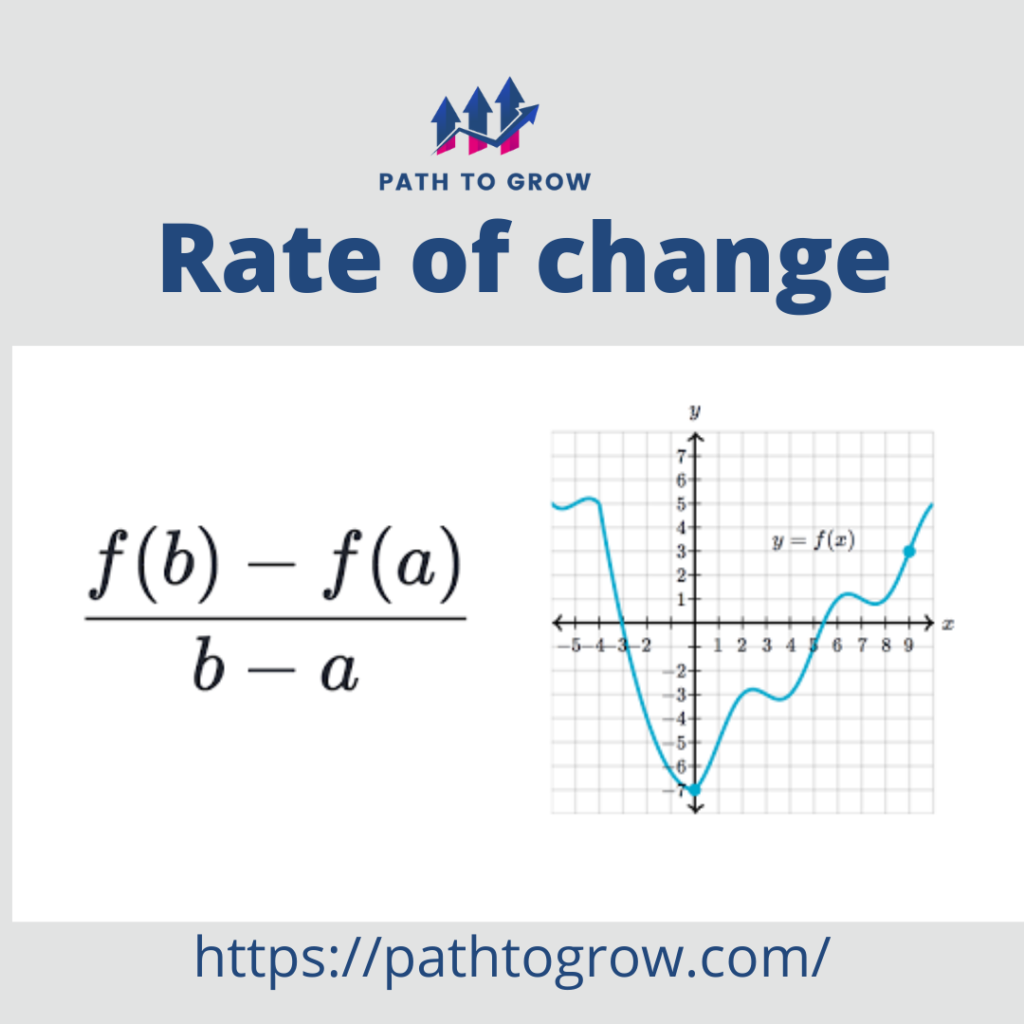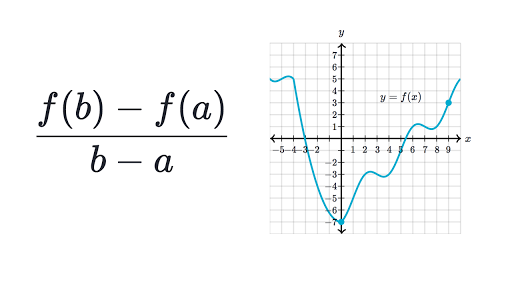# What is the Rate Of Change Formula? – Calculation and Application

## What is the Rate Of Change Formula? – Calculation and Application

The rate of change function is calculated by dividing the amount of change in one item with respect to another. So, it can be defined as the amount of change in a quantity with respect to another. The rate of change formula can be useful to deprive multiple possibilities in trading. Let us have a look in detail at the rate of change formula and how to calculate it.

## What is the rate of change formula?

The rate of change can be described as how one entity changes with respect to the change in the other entity. The formula to calculate the rate of change formula can be calculated for X and Y Coordinates can be described as Δy/ Δx = (y2 – y1 )/ (x2 – x1 ). In the Linear function, slope-intercept form is used to represent the rate of change for a line: y=mx+b. However, the rate of change for other functions is derived from the formula : (f(b)-f(a))/ b-a.

### Different Formula For Rate Of Change

• Formula 1: The basic formula for the rate of change is:

Rate of change = (Change in quantity 1) / (Change in quantity 2)

• Formula 2: Formulas of the rate of change in algebra

Δy/ Δx = y2−y1/x2−x1

• Formula 3: Rate of change of functions

(f(b)-f(a))/ b-a## Uses Of Rate Of Change Formula

As we have already discussed above, the rate of change formula helps us to understand the rate of change for a quantity with respect to another quantity. Here we have listed some of the applications of the rate of change formula.

• In a certain amount of time, the distance is covered by a car or any other mode of transport.
• The amount of current passes through an electric circuit that increases in amperes with respect to an increase in the volt of the circuit.
• For traders and investors, (read the difference between trading vs investing here.) it can also be used to detect secure momentum and trends in the trading.
• A number of people are required to perform a specific task or work.
• Work done per unit time.

## Relationship between rate of change and Price

The rate of change is mostly used to measure the change in a security’s price over the time. This is also known as the price rate of change (ROC). The price rate of change can be derived by taking the price of a security at time X minus the price of the same security at time A and dividing that result by the price at time Y.

Price ROC=(X−Y)/Y×100

where: X=price at current time

Y=price at previous time

## Conclusion

That is all that you need to know about the rate of change formula. The different application of the formula makes it quite useful in every sector. If you are a trader or investor then you can apply this formula to understand the chart or trends of different stocks with respect to the previous performance of the stocks. In Case, if you need any kind of assistance or help then you can contact us in the comment section.

1.  What Is the Formula for Rate of Change in Maths?

Ans: The formula for rate of change is: ROC = (Change in quantity 1) / (Change in quantity 2). It helps to define the rate of change in a quantity with respect to another.

2. What Is the Average Rate of Change Formula?

Ans: To Calculate the average rate, divide the total change occurring by the total time taken for it. The average velocity of an object is calculated using the same formula.

3. What Is the Instant Rate of Change Formula?

Ans: The rate or amount of change at an instant time or event is considered as the instantaneous rate of change formula. For a graph, the instantaneous rate of change at a specific point is the same as the tangent line slope.